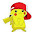### Guide to Solving Maximal Subarray Problem using Kadane's Algorithm

What is 'Maximal Subarray Problem'(MSP)?

Given an array having both positive and negative numbers, we need to find a continuous part of the array whose elements when added gives the largest possible sum.

1. consider the array [-1, 2, -1, 3]

Now, here is a list of all the possible subarrays along with the sum of their elements:

ELEMENTS SUM start index end index
-1 -1 0 0
-1, 2 1 0 1
-1, 2,-1 0 0 2
-1, 2,-1, 3 3 0 3
2 2 1 1
2,-1 1 1 2
2,-1, 3 4 1 3
-1 -1 2 2
-1, 3 2 2 3
3 3 3 3

From, the above table, its obvious that the answer is [ 2,-1, 3].
All other subarrays sum up to a value that is less than 4.

2. consider the array [-1,-2]

Here, the answer would be [-1]

3. consider the array [ 1, 2, 3]

Here the answer would be [ 1, 2, 3]

I hope you got the idea of what is 'Maximal Subarray Problem' by now.

Given an array, how can we find the MSP?

One way to find the MSP for a given array is to check out all the possible subarrays and thus find the subarray with maximal sum. This is called Brute-force approach as you are trying out each and every possibility to find an answer. You can implement it by using 2 for loops(nested).

Here's the pseudocode for Brute-force approach:

The time complexity of this approach is O(n3).

Now  Brute-force is not an efficient method to solve this problem. In fact, the MSP can be solved in linear time i.e, O(n). Kadane's algorithm is an O(n) method to solve the MSP.

Kadane's algorithm begins with a simple inductive question:

if we know the maximum subarray sum ending at position 'i', what is the maximum subarray sum ending at position 'i+1'?
The answer turns out to be relatively straightforward:
Either the maximum subarray sum ending at position 'i+1' includes the maximum subarray sum ending at position 'i', or it doesn't.
To put it another way:
The maximal subarray at position 'i + 1' will either be maximal subarray till position 'i' along with element at position 'i + 1' (or) element at position 'i + 1' alone.

maximal subarray till 'i+1'  =   maximum( maximal subarray till i + arr[i + 1],  arr[i + 1])
Thus, we can compute the maximum subarray sum ending at position 'i' for all positions 'i' by iterating once over the array. As we go, we simply keep track of the maximum sum we've ever seen.

Here's a pseudocode to find maximal subarray using Kadane's algorithm:

Here's a C program to solve the MSP using Kadane's algorithm. This is slightly modified order to even keep track of the starting and ending indices of the maximal subarray.

The output when the executable was run:

I hope the post was helpful for you :)

1.what if when all the elements are negative in the second method

1.Yes it does work for all the negative numbers!

2.This is not correct, program for the below input, it doesn't work.

[mdudekul@den03cis ~]\$ ./a.out
Original array: : 10 -1 2 11
Maximal sub-array: : 10 -1 2 11
[mdudekul@den03cis ~]\$

For the above input, it has to be , {2, 11}

1.Hey! No {10, -1, 2, 11} is still the maximal-subarray because sum of elements is 22 where as in {2, 11} its just 13. NOTE: A maximal subarray can have negative numbers!

3.thanks for your information really good and very nice web design company in velachery